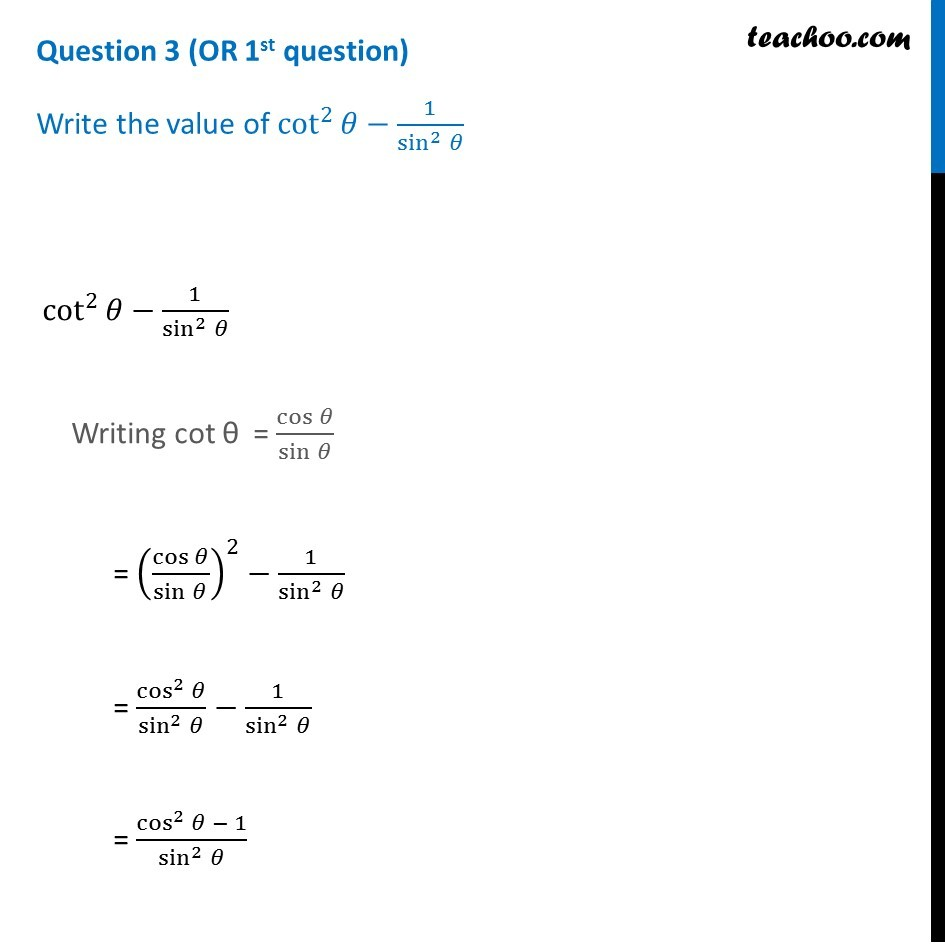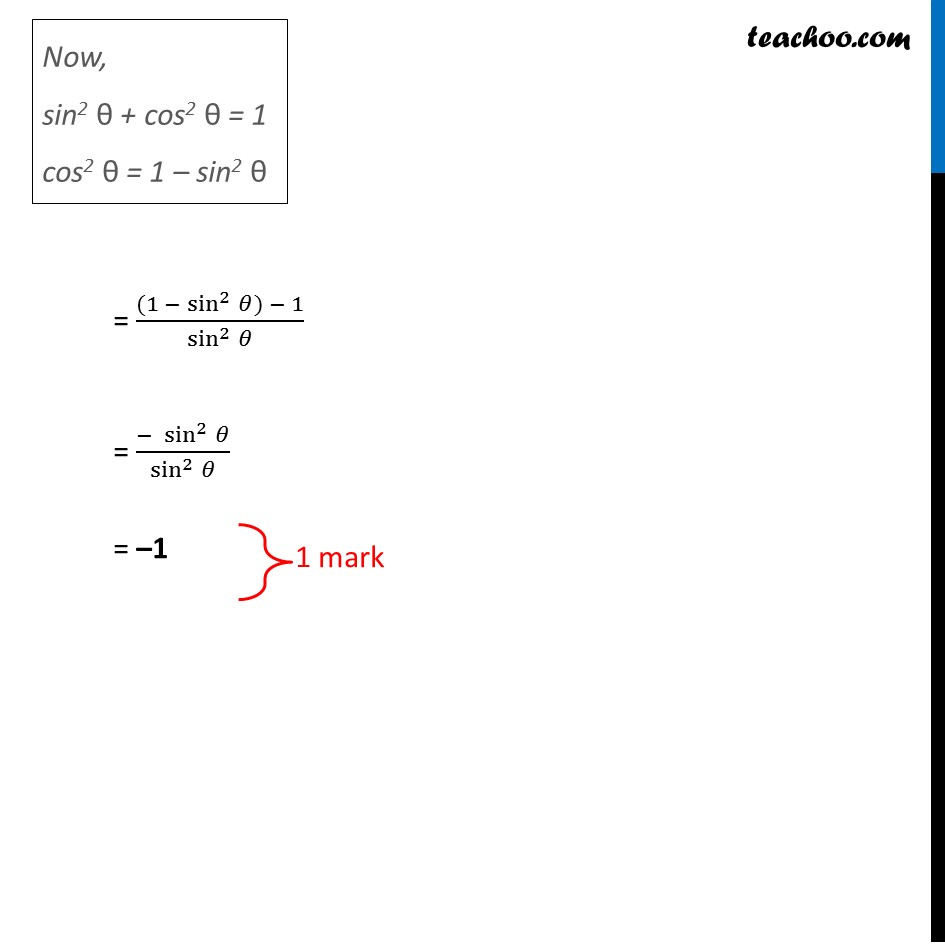Question 3 (OR 1 st question)

Write the value of cot 2 ⁡θ - 1/sin 2 ⁡θ1. Class 10
2. Solutions of Sample Papers for Class 10 Boards
3. CBSE Class 10 Sample Paper for 2019 Boards

Transcript

Question 3 (OR 1st question) Write the value of cot^2⁡𝜃−1/sin^2⁡𝜃 cot^2⁡𝜃−1/sin^2⁡𝜃 Writing cot θ = cos⁡𝜃/sin⁡𝜃 = (cos⁡𝜃/sin⁡𝜃 )^2−1/sin^2⁡𝜃 = cos^2⁡𝜃/sin^2⁡𝜃 −1/sin^2⁡𝜃 = (cos^2⁡𝜃 − 1)/sin^2⁡𝜃 Now, sin2 θ + cos2 θ = 1 cos2 θ = 1 – sin2 θ = ((1 − sin^2⁡𝜃) − 1)/sin^2⁡𝜃 = (− sin^2⁡𝜃)/sin^2⁡𝜃 = –1

CBSE Class 10 Sample Paper for 2019 Boards

Class 10
Solutions of Sample Papers for Class 10 Boards